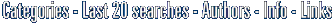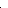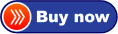# Visual Calculus Calculus grapher for pre-calculus calculus teaching and studying. greatO in short: ual Calculus is an easy-to-use calculus grapher for Graphing limit derivative function integral 3D vector series ode etc. Which can create 2D 2.5D and 3D function graphs animations aneasy

Easy software similar to Visual Calculus, about:
calculus grapher, calculus and pre calculusCategoriesVisual Calculus 3.9.2Click to enlarge

Description:
Visual Calculus is an easy-to-use calculus grapher for Graphing limit, derivative function, integral, 3D vector, double integral, triple integral, series, ode etc. Which can create 2D, 2.5D and 3D function graphs, animations and table graphs. 2D Features: explicit, implicit, parametric, and in equation; Cartesian and polar coordinate systems; curve and animation; graph of inverse function and derivative function; parity; maximum, minimum, and inflexion; integration; root; the length of curve; tangent and normal; curvature circle; intersection; line plot; scatter plot; stem plot; step plot; curve smoothing. 3D Features: cartesian, cylindrical, and spherical coordinate systems; 3D curve and surface; animation; 3D surface based on table data; surface by rotation; 3D vector; tangent and normal of 3D curve and surface; material and light. Ability to create color map, contour plot and vector plot. Ability to move, zoom in, zoom out and rotate graphs. Ability to save graphs as text file or bmp file. Ability to save animation as AVI file.
Price \$ 60 /Purchase Visual Calculus
Get it Now

Type: Demo
File size: 3837 Kb
Date: 06/03/2016
Homepage
Install support: Install and Uninstall
OS: Win2000, Win7 x32, Win7 x64, Win98, WinOther, WinServer, WinVista, WinVista x64, WinXP
System requirements:
Language: English
Recent changes in this Major Update:

Home and Education: Home and Education:Geometry and Mathematic Analysis

 FindGraphFindGraph is a graphing, curve-fitting, and digitizing tool for engineers, scientists and business. Discover the model that best describes your data.

 Visual CalculusVisual Calculus is an easy-to-use calculus grapher for Graphing limit, derivative function, integral, 3D vector, series, ode etc. Which can create 2D, 2.5D and 3D function graphs, animations and table graphs.

 Prime or notSearch, count, save and find primes then prove their primality.Using 4 tools:-The Primality Tester.-Mersenne numbers tester.-The Divisors finder.-The Search engine.-The convertor: Mersenne form-Fermat form-integer-The Splitter...

 Math-Flight 2Learning mathematics can be a challenge for anyone. Math Flight can help you master it with three fun activities to choose from! With lots of graphics and sound effects, your interest in learning math should never decline.

 Visual FractalWith this interesting fractal software, you can use Newton's method to solve a complex equation and show the fractal graph in the plot area.

 Visual ComplexVisual Complex is a graph software to create graph of complex function. 3D function graphs and 2D color maps can be created with this grapher.

 See above information and user's reviews about Visual Calculus Calculus grapher for pre-calculus calculus teaching and studying. great See also other good software tools: Home and Education: Home and Education:Geometry and Mathematic AnalysisSearch Soft14: Search: the Web within this Web site